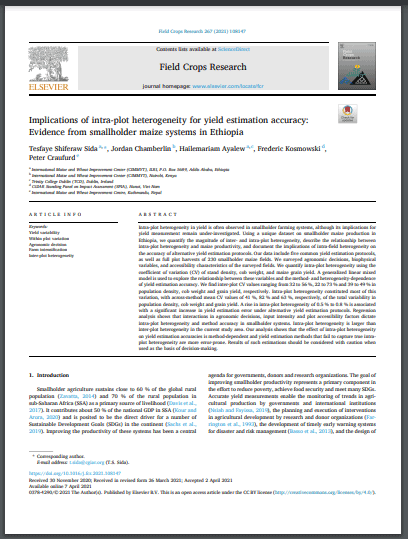# Implications of intra-plot heterogeneity for yield estimation accuracy: evidence from smallholder maize systems in EthiopiaShare this to :

Intra-plot heterogeneity in yield is often observed in smallholder farming systems, although its implications for yield measurement remain under-investigated. Using a unique dataset on smallholder maize production in Ethiopia, we quantify the magnitude of inter- and intra-plot heterogeneity, describe the relationship between intra-plot heterogeneity and maize productivity, and document the implications of intra-field heterogeneity on the accuracy of alternative yield estimation protocols. Our data include five common yield estimation protocols, as well as full plot harvests of 230 smallholder maize fields. We surveyed agronomic decisions, biophysical variables, and accessibility characteristics of the surveyed fields. We quantify intra-plot heterogeneity using the coefficient of variation (CV) of stand density, cob weight, and maize grain yield. A generalized linear mixed model is used to explore the relationship between these variables and the method- and heterogeneity-dependence of yield estimation accuracy. We find inter-plot CV values ranging from 32 to 56 %, 22 to 73 % and 39 to 49 % in population density, cob weight and grain yield, respectively. Intra-plot heterogeneity constituted most of this variation, with across-method mean CV values of 41 %, 82 % and 63 %, respectively, of the total variability in population density, cob weight and grain yield. A rise in intra-plot heterogeneity of 0.5 % to 0.8 % is associated with a significant increase in yield estimation error under alternative yield estimation protocols. Regression analysis shows that interactions in agronomic decisions, input intensity and plot accessibility factors dictate intra-plot heterogeneity and method accuracy in smallholder systems. Intra-plot heterogeneity is larger than inter-plot heterogeneity in the current study area. Our analysis shows that the effect of intra-plot heterogeneity on yield estimation accuracies is method-dependent and yield estimation methods that fail to capture true intra-plot heterogeneity are more error-prone. Results of such estimations should be considered with caution when used as the basis of decision-making.

Share this to :